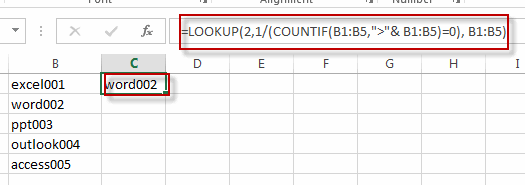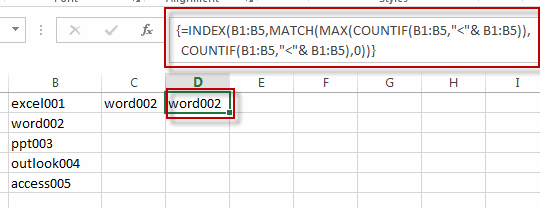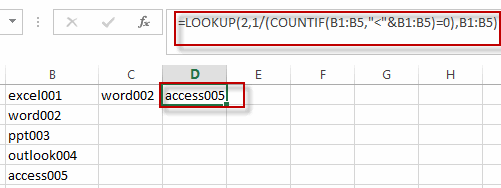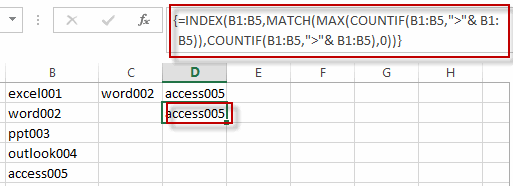# Finding the Max and Min value in an Alphanumeric Data

This post will guide you how to find the maximal and minimal value or string from an alphanumeric data list in excel. How do I retrieve or get the max or min string value based on alphabetic order from a string data in excel.

For example, if you want to find the Maximal or minimal string value from an alphanumeric data list in the range B1:B5, you can create a formula based on the LOOKUP function and the COUNTIF function.

To get the max value based on the alphabetic order, you can use the following formula:

`=LOOKUP(2,1/(COUNTIF(B1:B5,">"& B1:B5)=0), B1:B5)`You can also use the following array formula to achieve the same result. Just follow these steps to apply the array formula:

#1 type the formula in the formula box of cell D1

=INDEX(B1:B5,MATCH(MAX(COUNTIF(B1:B5,”<“& B1:B5)),COUNTIF(B1:B5,”<“& B1:B5),0))

#2 Press Ctrl + Shift +Enter on your keyboard to make the formula as an array formula.

#3 the formula will be change as the following style:

`{=INDEX(B1:B5,MATCH(MAX(COUNTIF(B1:B5,"<"& B1:B5)),COUNTIF(B1:B5,"<"& B1:B5),0))}`

#4 let’s see the result.To get the min value based on the alphabetic order, you can use the following formula:

`=LOOKUP(2,1/(COUNTIF(B1:B5,"<"&B1:B5)=0),B1:B5)`Of course, you can also use another array formula to get the minimal value based on alphabetic order, just refer to the above steps to apply the following array formula.

`{=INDEX(B1:B5,MATCH(MAX(COUNTIF(B1:B5,">"& B1:B5)),COUNTIF(B1:B5,">"& B1:B5),0))}`### Related Functions

• Excel LOOKUP function
The Excel LOOKUP function will search a value in a vector or array.The syntax of the LOOKUP function is as below:= LOOKUP (lookup_value, lookup_vector, [result_vector])…
• Excel COUNTIF function
The Excel COUNTIF function will count the number of cells in a range that meet a given criteria. This function can be used to count the different kinds of cells with number, date, text values, blank, non-blanks, or containing specific characters.etc.= COUNTIF (range, criteria)…
• Excel INDEX function
The Excel INDEX function returns a value from a table based on the index (row number and column number)The INDEX function is a build-in function in Microsoft Excel and it is categorized as a Lookup and Reference Function.The syntax of the INDEX function is as below:= INDEX (array, row_num,[column_num])…
• Excel MATCH  function
The Excel MATCH function search a value in an array and returns the position of that item.The MATCH function is a build-in function in Microsoft Excel and it is categorized as a Lookup and Reference Function.The syntax of the MATCH function is as below:= MATCH  (lookup_value, lookup_array, [match_type])….
• Excel MAX function
The Excel MAX function returns the largest numeric value from the numbers that you provided. Or returns the largest value in the array.= MAX(num1,[num2,…numn])…